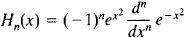# Hermite Polynomials

(redirected from Hermite polynomial)

## Hermite polynomials

[er′mēt ‚päl·ə′nō·mē·əlz]
(mathematics)
A family of orthogonal polynomials which arise as solutions to Hermite's differential equation, a particular case of the hypergeometric differential equation.

## Hermite Polynomials

a special system of polynomials of successively increasing degree. For n = 0,1, 2,..., the Hermite polynomials Hn (x) are defined by the formulaIn particular, H0 = 1, H1 = 2x, H2 = 4x2 – 2, H3 = 8x3 – 12x, and H4 = 16x4 – 48x2 + 12.

Hermite polynomials are orthogonal on the entire x-axis with respect to the weight function

e –x2

(seeORTHOGONAL POLYNOMIAL). They satisfy the differential equation

y″ – 2xy′ + 2ny = 0

and the recursion formulas

Hn+1 (x ) – 2xHn (x ) + 2nHn-1 (x ) = 0

H′n(x ) – 2nH–1(x ) = 0

Also sometimes called Hermite polynomials are polynomials that differ from those given above by certain factors dependent on n; sometimes

e–x2/2

is used as the weight function. The basic properties of the system were studied by P. L. Chebyshev in 1859 and C. Hermite in 1864.

References in periodicals archive ?
The associated function to s(x) on successive intervals [[x.sub.i-1], [x.sub.i]], 1 [less than or equal to] i [less than or equal to] n, with knots from [DELTA], is defined by [s.sub.i](x), that is, a(m - 1)-times continuously differentiable piecewise Hermite polynomial of degree 2m - 1, on I.
where [mathematical expression not reproducible] indicates nth order Hermite polynomial and [??] = (t - [T.sub.f])/[sigma] is the time variable.
(iv) Hermite polynomial with the Gaussian distribution is defined on (-[infinity], + [infinity]).
where [w.sub.0] are the waist width of Hermite-Gaussian beams, [H.sub.m](*) is a Hermite polynomial.
All seven methods use piecewise Hermite polynomial interpolants of nodal values, gradients, and, in the case of the first four methods, second partial derivatives.
When the input uncertainty obeys Gauss distribution, the basis function is the multidimensional Hermite polynomial.
Here, the pth order Hermite basis function is defined using the pth order Hermite polynomial as follows:
with real parameters [[sigma.sub.w], [[mu].sub.w] and the nth Hermite polynomial [h.sub.n].
Pal-type weighted (0, 2; 0) -interpolation on the zeros of Hermite polynomials. Let [{[x.sub.i]}.sup.n.sub.i=1] and [{[[bar.x].sub.i]}.sup.n-1.sub.i=1] be the zeros of [H.sub.n] and [H'.sub.n], respectively, where [H.sub.n] denotes the Hermite polynomial of degree n, for which
(a) approximate u(x) by the Hermite polynomial interpolant p(x) that satisfies the boundary conditions (31);
where x, x' [member of] R and the m-th Hermite polynomial is denoted as [H.sub.m](x) = [(-1).sup.m] [MATHEMATICAL EXPRESSION NOT REPRODUCIBLE IN ASCII] for any m [member of] No.

Site: Follow: Share:
Open / Close